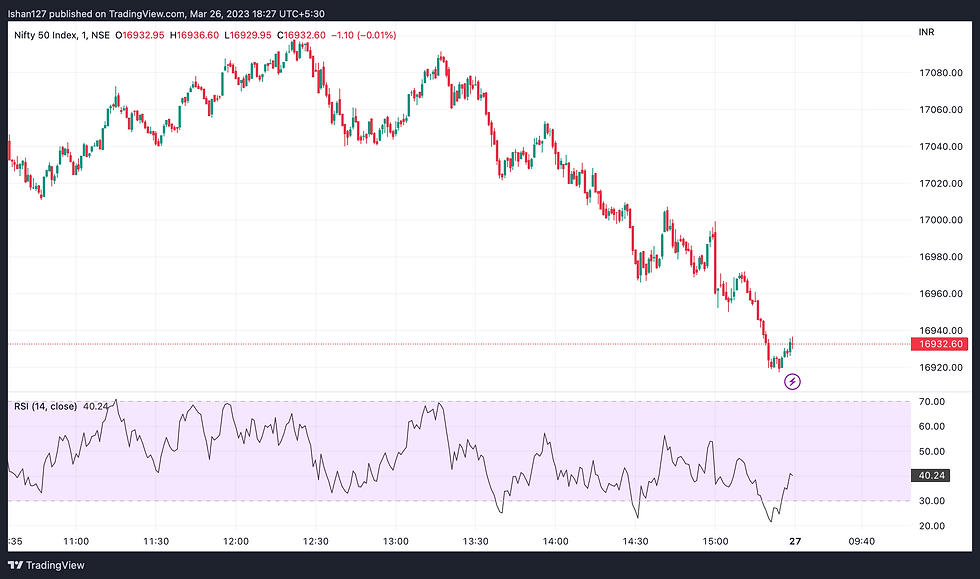top of page
Search

# How to use the Relative Strength Index (RSI) as a Momentum Indicator.

Updated: Apr 4

Relative Strength Index (RSI) is a popular technical indicator used by traders to measure the momentum of a stock or asset. Momentum trading involves buying or selling securities based on the speed or strength of the price movement. By analyzing the RSI, traders can identify potential trading opportunities and make informed decisions about their investments. In this blog post, we will discuss how to use the Relative Strength Index (RSI) as a momentum indicator and its significance in trading.## What is the Relative Strength Index (RSI)?

The Relative Strength Index (RSI) is a technical analysis tool used to measure the strength of an asset's price action. It compares the magnitude of a security's recent gains to the magnitude of its recent losses and plots the result on a scale from 0 to 100.

The RSI is calculated using the following formula:

RSI = 100 - [100 / (1 + RS)]

Where RS = Average Gain / Average Loss

The RSI oscillates between 0 and 100, with values above 70 indicating overbought conditions and values below 30 indicating oversold conditions. Traders use these levels as potential trading signals, buying when the RSI is oversold and selling when it is overbought.

## Using the Relative Strength Index (RSI) as a momentum indicator

The RSI can also be used as a momentum indicator, identifying potential changes in trend and momentum. Traders use the RSI to identify bullish or bearish divergences between the price and the indicator. A bullish divergence occurs when the RSI forms higher lows while the price forms lower lows, indicating that the momentum is shifting to the upside. Conversely, a bearish divergence occurs when the RSI forms lower highs while the price forms higher highs, indicating that the momentum is shifting to the downside.

Traders can also use the RSI to identify potential trend reversals by looking for overbought or oversold conditions. When the RSI reaches overbought levels above 70, it may signal that the asset is due for a correction or reversal to the downside. Conversely, when the RSI reaches oversold levels below 30, it may signal that the asset is oversold and due for a reversal to the upside.

It's important to note that the RSI is just one tool among many used in technical analysis. It should be used in conjunction with other indicators and analysis methods to make informed trading decisions.

## Conclusion

The Relative Strength Index (RSI) is a powerful technical indicator used by traders to measure the strength of an asset's price action. By using the RSI as a momentum indicator, traders can identify potential changes in trend and momentum and make informed trading decisions. It's important to use the RSI in conjunction with other indicators and analysis methods to get a full picture of the market.